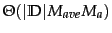2 views

In an extract from my textbook, it says that reducing the value of K when running this algorithm actually increases the complexity as it has to run more “smoothing”.

Can anyone explain this to me?

My understanding is that in 1NN, you feed it your training set. You test on your testing set. Assume your testing set has one point in it. It finds the one point closest to it in the training set and returns the value of this.

Surely this is less complex than finding the 3 closest points in 3NN, adding their values and dividing by three?

What have I misunderstood or overlooked?

by (108k points)

Training a kNN classifier simply consists of determining k and preprocessing documents. If we preselect some value for k and do not preprocess it, then kNN requires no training at all. In practice, we have to perform preprocessing steps like tokenization. It makes more sense to preprocess the training documents once as part of the training phase rather than repeatedly every time we classify a new test document.

Test time isfor kNN. It has a linear size of the training set as we need to compute the distance of each training document from the test document. Test time is independent of the number of classes J. kNN, therefore, has a potential advantage for problems with large J.

In short:

large value of K = simple model = underfit = low variance & high bias

Small vlaue of  K = complex model =overfit = high variance& low bias

If you wish to know more about K-means Clustering then visit this K-means Clustering Algorithm Tutorial.